﻿ Multiple Zeros, Non-Holomorphic Functions, Infinite IntervalsISSAC 94 - Symbolic-Numeric Nonlinear Equation Solving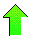Contents News View

 Multiple Zeros, Non-Holomorphic Functions, Infinite Intervals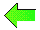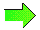Hoenders and Slump describe a method for determining number and multiplicities of zeros of a function based on a numerical quadrature technique, but as they show in their Tables 4-5, this technique is unstable.  It seems unlikely to us that any numerical technique could decisively solve this problem.  Symbolic methods can come to our aid in some instances.  First, if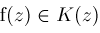is a rational function over a suitable computable extension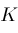of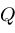, then square-free factorization is applicable.  Second, if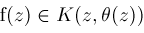where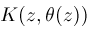is a purely transcendental extension of a computable extensionof,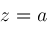is a multiple zero, and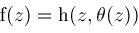where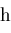is a rational function, then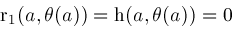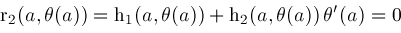are rational functions in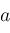and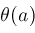identical to zero.  Since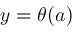is a common root of both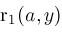and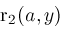, the resultant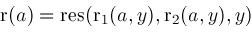must be zero.  Henceis a root of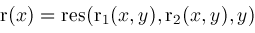and is algebraic over.  The equation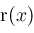can be solved symbolically for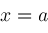and then this solution substituted into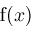and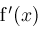to ascertain if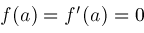provingis a multiple zero.  Third, if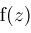can be decomposed as a composition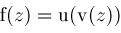,is a root of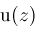with multiplicity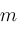,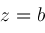is a root of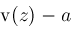with multiplicity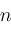, thenis a root ofwith multiplicity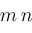A piecewise holomorphic function defined by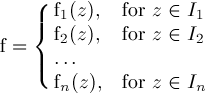consisting of finitely many holomorphic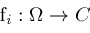may be treated asseparate inputs to our algorithm.

An expressioncontaining non-holomorphic subexpressions can sometimes be rewritten to become holomorphic or piecewise holomorphic.  As an example, suppose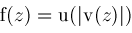where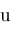and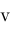are holomorphic.  Then solve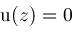and replace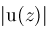by better expressions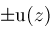Our algorithm is restricted to a finite interval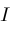.  In some cases, such as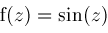, this is necessary, for otherwise there would be an infinite number of solutions.  In other cases, such as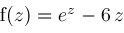, there are only finitely many solutions even on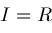.  The strategy proposed in this case is to use some asymptotic analysis to find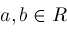such thatis non-zero on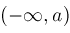and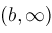leaving only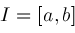to contend with.